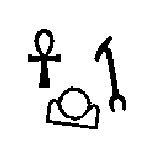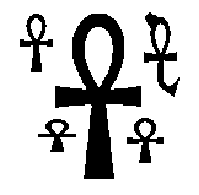# Programming contests

September 16, 2014 4:00 PM – December 21, 2014 8:00 PM

# Ancient Messages

In order to understand early civilizations, archaeologists often study texts written in ancient languages. One such language, used in Egypt more than 3000 years ago, is based on characters called hieroglyphs. Figure 1 shows six hieroglyphs and their names. In this problem, you will write a program to recognize these six characters.Figure 1: Six hieroglyphs

## Input Specification

The input consists of several test cases, each of which describes an image containing one or more hieroglyphs chosen from among those shown in Figure 1. The image is given in the form of a series of horizontal scan lines consisting of black pixels (represented by 1) and white pixels (represented by 0). In the input data, each scan line is encoded in hexadecimal notation. For example, the sequence of eight pixels `10011100` (one black pixel, followed by two white pixels, and so on) would be represented in hexadecimal notation as `9c`. Only digits and lowercase letters `a` through `f` are used in the hexadecimal encoding. The first line of each test case contains two integers, H and W. H (0 < H ≤ 200) is the number of scan lines in the image. W (0 < W ≤ 50) is the number of hexadecimal characters in each line. The next H lines contain the hexadecimal characters of the image, working from top to bottom. Input images conform to the following rules:

• The image contains only hieroglyphs shown in Figure 1.
• Each image contains at least one valid hieroglyph.
• Each black pixel in the image is part of a valid hieroglyph.
• Each hieroglyph consists of a connected set of black pixels, and each black pixel has at least one other black pixel on its top, bottom, left, or right side.
• The hieroglyphs do not touch, and no hieroglyph is inside another hieroglyph.
• Two black pixels that touch diagonally will always have a common touching black pixel.
• The hieroglyphs may be distorted, but each has a shape that is topologically equivalent to one of the symbols in Figure 1. (Two figures are topologically equivalent if each can be transformed into the other by stretching without tearing.)

The last test case is followed by a line containing two zeros.

## Output Specification

For each test case, display its case number followed by a string containing one character for each hieroglyph recognized in the image, using the following code:

Ankh: `A`
Wedjat: `J`
Djed: `D`
Scarab: `S`
Was: `W`
Akhet: `K`

In each output string, print the codes in alphabetic order. Follow the format of the sample output.

The sample input contains descriptions of test cases shown in Figures 2 and 3. Due to space constraints, not all of the sample input can be shown on this page.Figures 2 and 3: Test cases in the sample input

## Sample Input

1. `100 25`
2. `0000000000000000000000000`
3. `0000000000000000000000000`
4. `...(50 lines omitted)...`
5. `00001fe0000000000007c0000`
6. `00003fe0000000000007c0000`
7. `...(44 lines omitted)...`
8. `0000000000000000000000000`
9. `0000000000000000000000000`
10. `150 38`
11. `00000000000000000000000000000000000000`
12. `00000000000000000000000000000000000000`
13. `...(75 lines omitted)...`
14. `0000000003fffffffffffffffff00000000000`
15. `0000000003fffffffffffffffff00000000000`
16. `...(69 lines omitted)...`
17. `00000000000000000000000000000000000000`
18. `00000000000000000000000000000000000000`
19. `0 0`
1. `Case 1: AKW`
2. `Case 2: AAAAA`